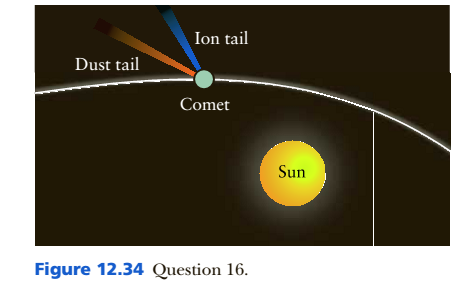Chapter 12, Problem 16Q

Chapter
Section
Textbook Problem

(Indicates a review question, which means it requires only a basic understanding of the material to answer. Questions without this designation typically require integrating or extending the concepts presented thus far.). Figure 12.34 shows the trajectory of a comet passing near the Sun. Describe how Newton would explain the deviation in the comet’s path from a straight line. Repeat the explanation as it might be given by Einstein.To determine

To describe:

How Newton's and Einstein would explain the deviation in the comet's path from a straight line.

Explanation

According to Newton, the force exerted by Sun’s radiation pressure and wind makes comet tails and due to pressure comet’s tail is away from the Sun. As a comet approaches the Sun, solar heating starts to vaporize the ices, releasing gas that forms a diffuse luminous sphere, called the coma around the comet nucleus. There is extra pressure from the Sun cause the deviation from the straight line. Einstein realized that massive objects caused a distortion in space-time...

Still sussing out bartleby?

Check out a sample textbook solution.

See a sample solution

The Solution to Your Study Problems

Bartleby provides explanations to thousands of textbook problems written by our experts, many with advanced degrees!

Get Started